×
Get Full Access to WSU - CHM 1220 - Class Notes - Week 6
Get Full Access to WSU - CHM 1220 - Class Notes - Week 6

×

WSU / Chemistry / CHM 1220 / What are the different properties of liquids?

# What are the different properties of liquids? Description

Feb 15 new chapter ­ EXAM 3

## What are the different properties of liquids?1. Comparison of Gases,Liquids,and Solids

Changes of State

2. Phase Transitions

3. Phase Diagrams

Liquid State

4. Properties of Liquids: Surface Tension and Viscosity

5. Intermolecular Forces: Explaining Liquid Properties

Solid State

6. Classification of Solids by Type of Attraction of Units

7. Crystalline Solids: Crystal Lattices and Unit Cells

8. Structures of Some Crystalline Solids

9. Calculations Involving Unit­Cell Dimensions

10.Determining Crystal Structure by X­Ray Diffraction

Learning Objectives

1. Comparison of Gases, Liquids, and Solids

a. Recall the definitions of gas,liquid,and solid given in Section 1.4. b. Compare a gas, a liquid, and a solid using a kinetic molecular theory description.

## What is the dipole moment?c. Recall the ideal gas law and the van der Waals equation for gases (there are no similar simple equations for liquids and solids).

2.Changes of State

Phase Transitions

a. Define change of state (phase transition)

b. Define melting,freezing,vaporization, sublimation, and condensation.Don't forget about the age old question of What are the three types of legitimate authority?
If you want to learn more check out How does functional magnetic resonance imaging work?

c. Define vapor pressure.

d. Describetheprocessofreachingadynamic equilibrium that involves the vaporization of a liquid and condensation of its vapor.

e. Describe the process of boiling.

f. Define freezing point and melting point.

g. Define heat (enthalpy) of fusion and heat (enthalpy) of vaporization. h. Calculate the heat required for a phase change of a given mass of substance.

## What is the vapor pressure?i. Describe the general dependence of the vapor pressure (ln P) on the temperature (T). j.State the Clausius–Clapeyron equation (the two­point form).

k.Calculate the vapor pressure at a given temperature.

l.Calculate the heat of vaporization from vapor pressure.

3.Phase Diagrams

a. Define phase diagram.

b. Describe the melting­point curve and the vapor­pressure curves (for the liquid and the solid) in a phase diagram. If you want to learn more check out Are there meaningful differences across developed democracies?

c. Define triple point.

d.Define critical temperature and critical pressure

e. Relate the conditions for the liquefaction of a gas to its critical temperature. Liquid State

4. Properties of Liquids; Surface Tension and Viscosity

a. Define surface tension.

b. Describe the phenomenon of capillary rise

c. Define viscosity.

5. Intermolecular Forces; Explaining Liquid Properties

a. Define intermolecular forces.

b. Define dipole–dipole force.

c.Describe the alignment of polar molecules in a substance.

d.Define London(dispersion) forces.

e.Note that London forces tend to increase with molecular mass. f. Relate the properties of liquids to the intermolecular forces involved. g. Define hydrogen bonding.

h. Identify the intermolecular forces in a substance.

i. Determine relative vapor pressures on the basis of intermolecular attractions.

7. Crystalline Solids; Crystal Lattices and Unit Cells

a. Define crystalline solid and amorphous solid.

b.Define crystal lattice and unit cell of a crystal lattice. If you want to learn more check out What was the nickname for the united fruit company?

c. Define simple cubic unit cell, body­centered cubic unit cell, and face­centered cubic unit cell. If you want to learn more check out What are the five largest countries by population?
If you want to learn more check out What is a moral life and why should i live it?

d. Determine the number of atoms in a unit cell.

e.Describe the two kinds of crystal defects.

8. Structures of Some Crystalline Solids

a. Define hexagonal close­packed structure and cubic close­packed structure. b. Define coordination number.

c. Note the common structures (face­centered cubic and body­centered cubic) of metallic solids.

d. Describe the three types of cubic structures of ionic solids.

e. Describe the covalent network structure of diamond and graphite. 9. Calculations Involving Unit­Cell Dimensions

a. Calculate atomic mass from unit­cell dimension and density.

b. Calculate unit­cell dimension from unit­cell type and density.

10. Determining Crystal Structure by X­Ray Diffraction

a. Describe how constructive and destructive interference give rise to a diffraction pattern.

b. Note that diffraction of x­rays from a crystal gives information about the positions of atoms in the crystal.

Comparing Gases, Liquids, and Solids

Gases are compressible fluids.

Liquids are relatively incompressible fluids.

Solids are nearly incompressible and rigid.

A change of state or phase transition is a change of a substance from one state (solid, liquid, gas) to another. The specific name or names for each of these transitions are given below.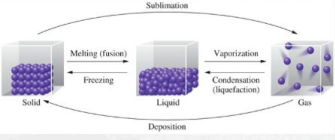The vapor pressure of a liquid at a particular temperature is the partial pressure of the vapor over the liquid measured at equilibrium.

When a liquid is placed in a closed vessel, the partial pressure of its vapor increases over time until it reaches equilibrium. At equilibrium, evaporation and condensation continue to occur, but do so at the same rate. This situation, which is called a dynamic equilibrium, is illustrated on the next slide.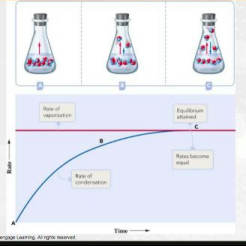The vapor pressure depends on the liquid and on the temperature. This relationship is illustrated on the next slide for four substances. Note that as temperature increases, vapor pressure increases.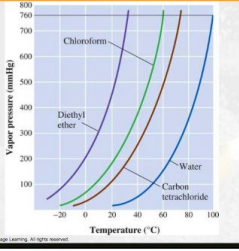The boiling point is the temperature at which the vapor pressure is equal to the pressure on the liquid, usually atmospheric pressure. At this temperature, bubbles of gas form within the liquid, as illustrated in the figure. The normal boiling point is measured at 1 atmosphere pressure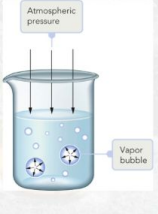When the pressure on the liquid increases, as is the case with a pressure cooker, the boiling point increases.

Conversely, when the pressure on the liquid decreases, as is the case at high altitude, the boiling point decreases.

The freezing point is the temperature at which a pure liquid changes to a crystalline solid (or freezes).

The melting point is the temperature at which a crystalline solid changes to a liquid (or melts).

Any change of state requires that energy be added to or removed from the system. As shown in the graph, for water, there are two regions that remain at the same temperature even as heat is added. Those represent regions of phase change.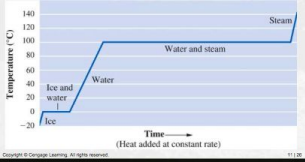­phase change means no temperature change

q=mct (there’s a specific heat for solid liquid gas each so it’s diff and the slope of each of those lines is diff)

­ACE regions (slope lines) use phase change

­BD use temp change

­We can learn about IMF by studying heats of vaporization, the energy needed to cause 1 mol of liquid to vaporize

­It takes energy to break apart molecules

­entropy drives the process, favors the process of solid to liquid to gas ­Polar molecules are attracted to each other

­Dipole moment: a quantitative measure of the degree​…?

­We can measure the energy that it takes to condense/vaporize a noble gas (1.77 kJ/mol and happens at ­246 degrees celsius)

­In a NP molecule, the charge is uniformly distributed over time. In any ONE instant however, the charge is not uniformly distributed. In that instant, there is an instantaneous dipole. (induced dipole ­ the one molecule that is polar in that instant INDUCES dipoles, and it averages out over time to make it NP) There is a net force of attraction called instantaneous dipole, aka London forces.

­LF are the weak attractive forces between molecules resulting from the small, instantaneous dipoles that occur because of the varying positions of the electrons during their motion about the nuclei

­more electrons, more atomic number ­­­> higher london force

­LF is proportional to the number of electrons, but WE use molar mass as an estimate. So look at it in terms of higher molar mass, higher LF

­More condensed structures give us weaker forces, more spread out like straight chains of carbon, give us a higher boiling point

­Heat of vaporization is the energy needed to change from liquid to vapor, without changing the temperature

­”Van der waals forces” includes both dip dip forces and london forces ­polar molecules exhibit dip dip forces that result in alignment of the molecules ­Higher boiling point doesn’t always mean higher LF, going down a group LF increases

February 17

­Vapor pressure ­ the vapor pressure of a liquid at a particular temperature is the partial pressure of the vapor over the liquid measured at EQ

­If the heat of vaporization is high, we expect a lower vapor pressure than if the heat of vaporization is low. While we cannot predict vapor pressures, we can compare them based on IMF. ­rate of evaporation = rate of condensation is the definition of EQ

­Boiling point is the temperature at which the vapor pressure = external pressure ­When the vapor pressure is 1 atm that is the normal

­As IMF forces increase, VP increases

­ln(P2/P1) = ­delta Hvap/R (1/t2 ­ 1/t1) (temperature in kelvin)

­The BP is the temperature at which the VP is equal to the pressure on the liquid. At this temperature, bubbles of gas form WITHIN the liquid. The normal BP is measured at 1 atm.

­We use boiling to purify. To lower the BP (because some compounds decompose before they boil), so decrease the pressure to lower BP

February 19

­A phase diagram is a graphic way to summarize the conditions under which the various states of a substances are stable. The states are separated by lines that show the equilibrium between the states.

­Normal BP is the temperature when the vapor pressure is 1 atm

­The critical point gives the temperature (critical temperature) at which the liquid state can no longer exist and the pressure at that temp (critical pressure). Above this temp and pressure, there is only one state (supercritical fluid.)

Chapter 5­ Gases

Page ExpiredIt looks like your free minutes have expired! Lucky for you we have all the content you need, just sign up here